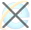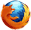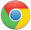HTML output for 属性

HTML <output> for 属性HTML <output> 标签

实例

<form oninput="x.value=parseInt(a.value)+parseInt(b.value)">0
<input type="range" id="a" value="50">100
+<input type="number" id="b" value="50">
=<output name="x" for="a b"></output>
</form>

浏览器支持Firefox、Opera、Chrome 和 Safari 浏览器都支持 for 属性。

定义和用法

for 属性规定计算中使用的元素与计算结果之间的关系。

HTML 4.01 与 HTML5之间的差异

<output> 标签是 HTML5 中的新标签。

语法

<output for="element_id">

属性值

element_id规定一个或多个元素的 id 列表，以空格分隔。这些元素描述了计算中使用的元素与计算结果之间的关系。HTML <output> 标签